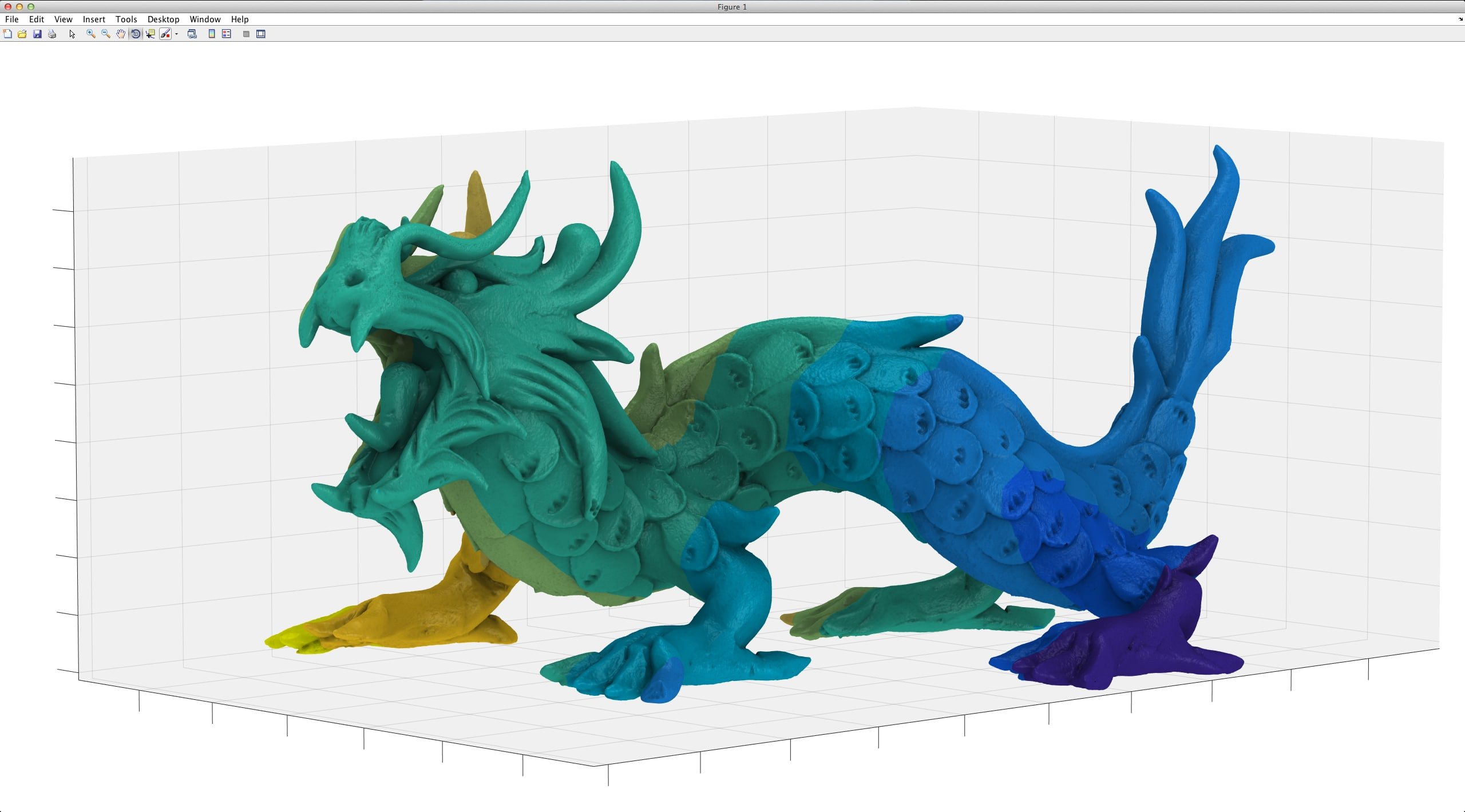## Matlab Project Center in Chennai

It is completely a Mathwork programming language. It is started with maths mainly into matrix programming language. It is a 4th generation high level programming language, the program also includes other programming languages like C, C++ & Java. It also has plenty of mathematical calculations in generating plots and other stuff. We deal with Matlab project in Chennai also we are one of the Best Matlab project centers in Chennai.

Plotting depends on 2D & 3D graphics

Linear Algebra

It uses Matrix

Mathematical Algebric functions

Numerical calculations

Analysis of data

Non linear functions

Statistics

And various other functions